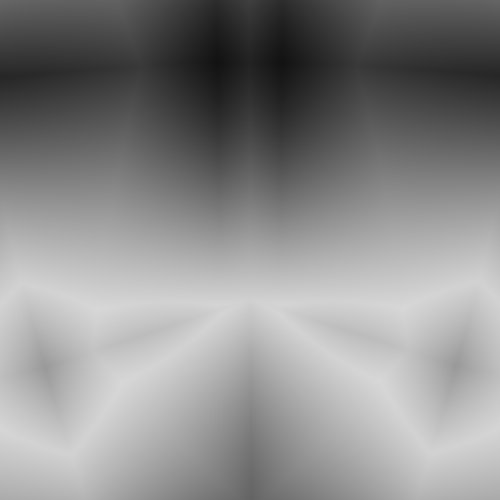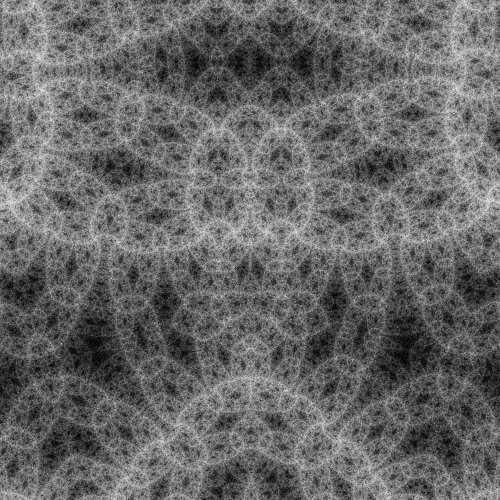### 20110218-1

2011, digital image and unique archival pigment print

Back to the index

An animated deep zoom into this algorithmic world can seen here. Check this blog post for more information.

March 19th 2011

## Piling Ducks

Today I would like to explain what the "Ducks" algorithm has to do with pattern piling. Recall that the Ducks algorithm looked very much like the algorithm drawing the Mandelbrot set. A transformation of the complex plane is iterated, and each point is colored using a property of its orbit. In the case of the Ducks fractal, the prescription to color the points was to perform the average of the norm of the points in the orbit, and turn this number into a color by mean of a linear color gradient. There are two ways of looking at this coloring method.

The method of coloring orbits using their distance to a shape (here a point at the origin) is known as an "orbit trap" method, and is a very well-known way of coloring the Mandelbrot set. An obvious way of generalizing the coloring of the Ducks fractal described above is to use other shapes. In practice, I didn't get extremely interesting results using other shapes. (See however this image, using a line as a shape instead of a point. The parallel curves that can be seen a bit everywhere are due to the use of a line.) Orbit trap algorithm usually color the point by using the distance of the closest point in the orbit. This method of coloring however has the unpleasant tendency of destroying the space-filling patterns. There are of course zillions of other twist that can be applied to the orbit trap algorithm, but I doubt they would yield extremely interesting results in the case of the Ducks space filling patterns.

The second way of looking at the coloring method described above is to split the mean by iteration. At each iteration, we modify the color of each point depending on its position. At the 0th iteration, the plane is not deformed, and we get simply a radial gradient. After the first iteration, as the points have moved non-trivially under the map, a more complicated pattern appears, that we add to the radial gradient. Similarly, we add the patterns corresponding to each iteration in order to get the final image. This is exactly pattern piling! In the standard version of pattern piling, the idea is to sum rescaled copies of a given pattern. In contrast, here the pattern is in some sense dynamically generated at each iteration. Below is an animated gif file showing eight patterns each separated by six iterations. (They are taken from 20110213-1).Eight patterns created by the iterations of the Ducks algorithm. There are six iterations between each successive patterns.

The characteristic size of the patterns tends to get smaller when the number of iterations increases, so there is no need to perform any resizing in order to get a space filling pattern. When we add these patterns, here is what we get.The eight patterns presented before are summed in turn.

Remark that interesting interferences appear between the patterns. The isolated patterns all displayed some prominent horizontal structure. However, these were alternating between a light and a dark color. Because of this, they disappear when they are summed.

I was really happy when I realized this relation to pattern piling, because it means that all the tricks that make pattern piling a versatile tool to create rich imagery can be applied to this algorithm as well. Just to give an example that was hinted at above, we are by no mean obliged to perform the mean on all iterations. Rather, we can skip a certain number of iterations at each steps (five in the example above). Because there are so many interferences between patterns, skipping some of them has dramatic effects on the final image. Here is the same fractal at the same location, but this time between zero to nine iterations were skipped.The patterns obtained by skipping zero to nine iteration at each step when performing the mean.

Remark that the horizontal structures that were interfering destructively when we were skipping five iteration appear beautifully when the number of skipped iterations is even. 20110212-1 was constructed with the same Ducks Julia set, but by skipping 1 iteration at each step to perform the mean. Needless to say that this vastly expands the already amazingly rich variety of patterns the Ducks algorithm is able to produce.

You can check the works using the Ducks algorithm here. Note that Mikael Hvidtfeldt Christensen, aka Syntopia, implemented the Ducks algorithm in his open fractal program, Fragmentarium. Check his pictures on Flickr. Happy exploring...